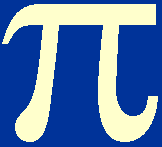Without even fully solving the homologous equations of stellar structure, we can deduce the gradients of the mass-luminosity and luminosity-effective temperature relations for main-sequence stars.

We have assumed that the luminosity of any point inside a star depends on some power of Ms but otherwise only on the fractional mass, m, i.e.:

L = Msa3 L*(m).

At the surface of the star (m = 1), this equation becomes:

Ls = Msa3 L*(1).

Since L*(1) is the same for all stars of the same chemical composition, i.e. it is a constant, we can write:

LsMsa3.

Hence we can see that the homologous stellar models predict a mass-luminosity relation in which the luminosity is proportional to the a3-th power of the mass. We will return later to the possible values of a3 and how this compares with the observed mass-luminosity relation.

Turning now to the luminosity-effective temperature relation, these two quantities are related to the radius of a star through the well-known relation:

Ls = 4rs2Te4.

Hence, we can write:

Lsrs2 Te4.

Combining this equation with the relations rs = Msa1 r*(1) and Ls = Msa3 L*(1), we obtain:

Msa3 L*(1)Ms2a1 r*(1)2Te4.

Since r*(1), like L*(1), is the same for all stars of the same chemical composition, i.e. it is a constant, we can rearrange the above equation as follows:

MsTe 4/(a3-2a1).

We have already proved that LsMsa3, so we can write:

LsTe 4a3/(a3-2a1).

This shows that stars lie on a straight line of gradient 4a3/(a3-2a1) in the theoretical HR diagram (a plot of log Ls versus log Te) and this might be identified with the main sequence.

We must now see if the predicted gradients of the mass-luminosity and luminosity-effective temperature relations are in agreement with the observed values. In order to prove that this is so, we must solve the 5 algebraic equations for a1 , a2 , a3 , a4 and a5 which we derived earlier:

I.          4a1 + a5 = 2,

II.          3a1 + a2 = 1,

III.         a3 = 1 + a2 +a4,

IV.         4a1 + (4-)a4 =a2 + a3 + 1,

V.          a5 = a2 + a4.

We have already stated that a general solution to these equations is too complicated to give here, but it is possible to write down solutions for special values of,and, which we will do now.

In our discussions of stellar opacity, we found that one approximation to the opacity law which appears to work well at intermediate temperatures is given by= 1 and= -3.5, i.e.=0/ T3.5.

A reasonable approximation to the rate of energy generation by the proton-proton chain is given by= 4, i.e.=0T4.

Substituting= 1,= -3.5 and= 4 into equations III and IV, we obtain a new, simplified set of equations to solve:

VI.          4a1 + a5 = 2,

VII.          3a1 + a2 = 1,

VIII.         a3 = 1 + a2 + 4a4,

IX.          4a1 + 7.5a4 = a2 + a3 + 1,

X.           a5 = a2 + a4.

We now have 5 algebraic equations with 5 unknowns, so it is a simple matter to obtain an exact solution by eliminating each of the a's in turn from the above equations until an expression for a3 is obtained. This expression can then be used to determine a1. Rather than follow through this (easy but tedious) part of the solution, we will simply state the results:

a3 = 71 / 13,   and

a1 = 1 / 13.

Substituting these values into the mass-luminosity and luminosity-effective temperature relations derived above, we obtain:

LsMs71/13,   and

LsTe 284/69.

The observed mass-luminosity law is not a simple power law but, if the central part of the curve (corresponding to stars of about solar mass) is approximated by a power law, it has an exponent of approximately 5, in good agreement with the value of 5.5 predicted by the above homologous solution; see figure 10.4 in Bohm-Vitense. Similarly, the lower part of the main-sequence on the observed luminosity-effective temperature (or HR) diagram is well represented by the power-law exponent of 4.1 predicted by the above homologous solution; see figure 10.5 in Bohm-Vitense. We have therefore verified the observed mass-luminosity relation of main-sequence stars and the slope of the main-sequence on the HR diagram and hence fulfilled one of our initial aims when we discussed the observed properties of stars at the start of this lecture course.